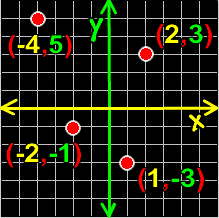# Online Math Dictionary: C

Easy to understand math definitions for K-Algebra mathematics
Just scroll down or click on the word you want and I'll scroll down for you!

 Cartesian  coordinates Cartesian plane central angle centroid of a triangle chord circle circumference circumscribed clockwise coefficient column combinations combinatorics commutative  property of addition commutative property of multiplication complementary angles complement of an angle complex numbers composite concave polygon cone congruent conjugate consecutive convex polygon coordinate coplanar corresponding sidesand angles counter-clockwise Cramer's Rule cube cubic equation cubic polynomial cuboctahedron cylinder

 Cartesian Coordinates(Read the definition below of Cartesian plane first) Coordinates on the Cartesian plane are a set of numbers officially called "an ordered pair" that are in the form ( x , y ) ...  The x guy is how far to the right or left you've counted over... and the y guy is how far up or down you've counted. For more info on graphing with Cartesian coordinates, check out my Plotting Points lesson.